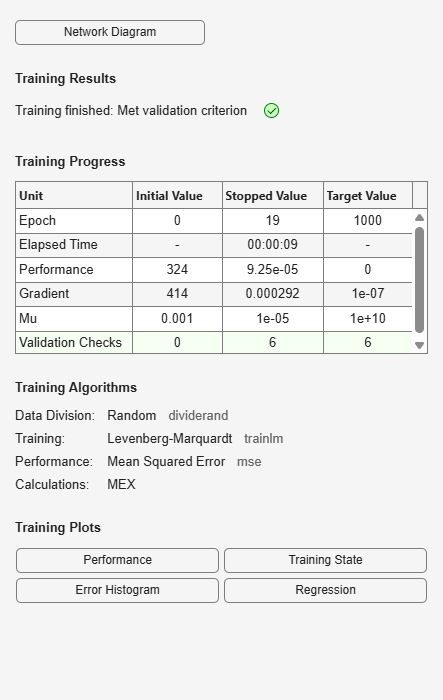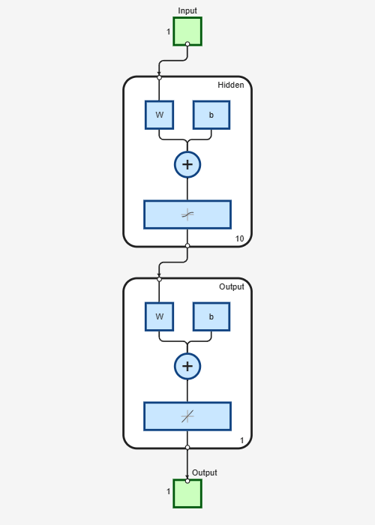# feedforwardnet

Generate feedforward neural network

## Syntax

``net = feedforwardnet(hiddenSizes,trainFcn)``

## Description

example

````net = feedforwardnet(hiddenSizes,trainFcn)` returns a feedforward neural network with a hidden layer size of `hiddenSizes` and training function, specified by `trainFcn`.Feedforward networks consist of a series of layers. The first layer has a connection from the network input. Each subsequent layer has a connection from the previous layer. The final layer produces the network’s output.You can use feedforward networks for any kind of input to output mapping. A feedforward network with one hidden layer and enough neurons in the hidden layers can fit any finite input-output mapping problem.Specialized versions of the feedforward network include fitting and pattern recognition networks. For more information, see the `fitnet` and `patternnet` functions.A variation on the feedforward network is the cascade forward network, which has additional connections from the input to every layer, and from each layer to all following layers. For more information on cascade forward networks, see the `cascadeforwardnet` function.```

## Examples

collapse all

This example shows how to use a feedforward neural network to solve a simple problem.

Load the training data.

`[x,t] = simplefit_dataset;`

The 1-by-94 matrix `x` contains the input values and the 1-by-94 matrix `t` contains the associated target output values.

Construct a feedforward network with one hidden layer of size 10.

`net = feedforwardnet(10);`

Train the network `net` using the training data.

`net = train(net,x,t);`View the trained network.

`view(net)`Estimate the targets using the trained network.

`y = net(x);`

Assess the performance of the trained network. The default performance function is mean squared error.

`perf = perform(net,y,t)`
```perf = 1.4639e-04 ```

## Input Arguments

collapse all

Size of the hidden layers in the network, specified as a row vector. The length of the vector determines the number of hidden layers in the network.

Example: For example, you can specify a network with 3 hidden layers, where the first hidden layer size is 10, the second is 8, and the third is 5 as follows: `[10,8,5]`

The input and output sizes are set to zero. The software adjusts the sizes of these during training according to the training data.

Data Types: `single` | `double`

Training function name, specified as one of the following.

Training FunctionAlgorithm
`'trainlm'`

Levenberg-Marquardt

`'trainbr'`

Bayesian Regularization

`'trainbfg'`

BFGS Quasi-Newton

`'trainrp'`

Resilient Backpropagation

`'trainscg'`

`'traincgb'`

Conjugate Gradient with Powell/Beale Restarts

`'traincgf'`

`'traincgp'`

`'trainoss'`

One Step Secant

`'traingdx'`

Variable Learning Rate Gradient Descent

`'traingdm'`

Gradient Descent with Momentum

`'traingd'`

Example: For example, you can specify the variable learning rate gradient descent algorithm as the training algorithm as follows: `'traingdx'`

For more information on the training functions, see Train and Apply Multilayer Shallow Neural Networks and Choose a Multilayer Neural Network Training Function.

Data Types: `char`

## Output Arguments

collapse all

Feedforward neural network, returned as a `network` object.

## Version History

Introduced in R2010b